# 变换要素

## 变换所选要素或图层

1. 编辑选项卡中，关闭拓扑，选择捕捉首选项，然后显示修改要素窗格。
1. 管理编辑组中，单击拓扑箭头，然后选择关闭拓扑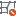2. 捕捉组中，单击捕捉下拉菜单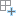，然后启用您的捕捉首选项。

要在编辑要素时暂时关闭捕捉，请按住空格键

3. 要素组中，单击修改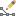2. 展开对齐并单击变换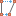3. 用以下方法之一选择要素：
• 要变换所选要素，请单击所选要素选项卡，然后单击选择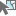并选择要素。
• 要优化选择，请在树视图中右键单击某个要素，然后单击取消选择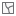仅选择此项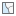• 要变换一个或多个图层上的所有要素，请单击图层选项卡并选择图层。
4. 单击变换方法下拉箭头，然后选择变换方法。
• 仿射 - 不同程度地缩放、旋转、平移、反映和倾斜要素。

此方法至少需要三个位移链接。

• 相似 - 均匀缩放、旋转、平移和反映要素。

5. 绘制适当数量的两点位移链接，以定义要变换的要素的起点和目的地位置。对于可绘制的链接数量没有限制。
##### 注：

当您绘制活动方法所需的最少位移链接时，RMS 误差将显示在窗格顶部。

1. 单击添加新链接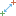线段构造工具栏显示在地图的底部。

2. 针对要变换的要素，捕捉边或折点的 起点。
3. 捕捉新目标位置的端点。
6. 要删除位移链接，请单击选择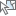，选择链接，然后按 Delete 键，或者单击鼠标右键并单击删除
• 要删除所有位移链接，请在窗格中单击删除所有链接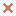7. 单击变换

将变换要素并自动删除位移链接。

## 从文件加载链接

``````3	1032507.603691	1865947.186470	1032507.207115	1865926.170407
2	1032508.350801	1865986.785187	1032509.079486	1866025.405116
1	1032388.666424	1865961.179459	1032361.009572	1865981.280533``````

## 均方根 (RMS) 误差

RMS 误差用于根据位移链接的数量和位置报告变换的整体适应度。每个链接会生成测量目的地控制点位置及其实际变换位置之间拟合的残差。

RMS 误差随着原始数据的精度和源地图的比例而变化。在某些情况下，高 RMS 误差可能表示您的数据将发生大量拉伸或倾斜。

## 等式

### 仿射

``````x’ = Ax + By + C
y’ = Dx + Ey + F``````

``A = Sx cos rB = (Sy / cos s) sin(s - r) C = DxD = Sx sin rE = (Sy / cos s) cos(s - r) F = DySx, Sy = Scaling factors (can be negative) Dx, Dy = Translation distances in x and y directions (can be negative) s = Skew angle of shearing along x-axis, measured from y-axis (-pi/2 < s < pi/2) r = Rotation angle in radians, measured counterclockwise from x-axis (- pi < r <= pi)``

### 相似

``````x' = Ax + By + C
y' = -Bx + Ay + F``````

``````A = s * cos tB = s * sin tC = Translation in x direction F = Translation in y direction
s = Scale change (same in x and y directions) t = Rotation angle, measured counterclockwise from the x-axis``````

### 射影

``x' = (Ax + By + C) / (Gx + Hy + 1) y' = (Dx + Ey + F) / (Gx + Hy + 1)``